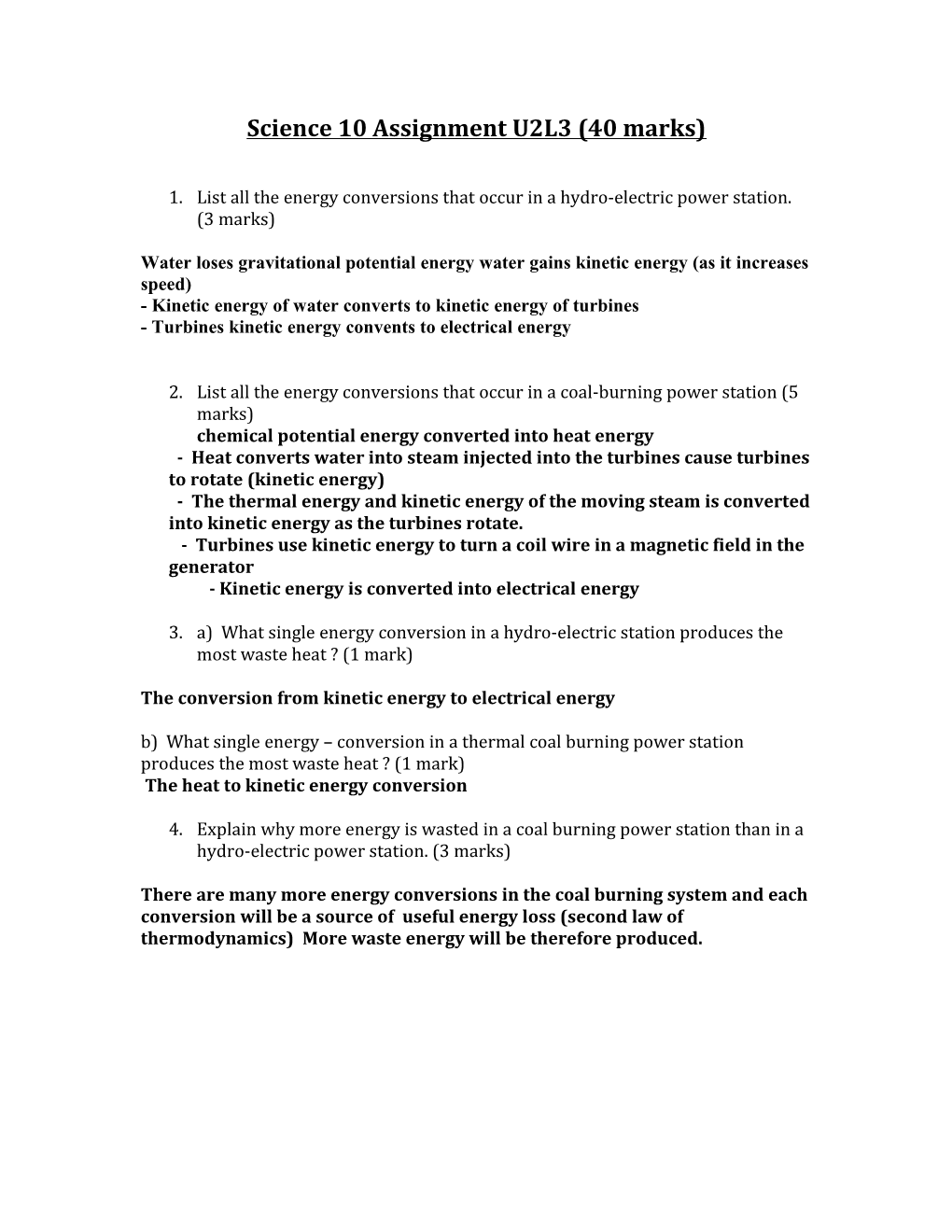# Water Loses Gravitational Potential Energy Water Gains Kinetic Energy (As It Increases Speed)Science 10 Assignment U2L3 (40 marks)

1. List all the energy conversions that occur in a hydro-electric power station. (3 marks)

Water loses gravitational potential energy water gains kinetic energy (as it increases speed)

- Kinetic energy of water converts to kinetic energy of turbines

- Turbines kinetic energy convents to electrical energy

1. List all the energy conversions that occur in a coal-burning power station (5 marks)

chemical potential energy converted into heat energy

- Heat converts water into steam injected into the turbines cause turbines to rotate (kinetic energy)

- The thermal energy and kinetic energy of the moving steam is converted into kinetic energy as the turbines rotate.

- Turbines use kinetic energy to turn a coil wire in a magnetic field in the generator

- Kinetic energy is converted into electrical energy

1. a) What single energy conversion in a hydro-electric station produces the most waste heat ? (1 mark)

The conversion from kinetic energy to electrical energy

b) What single energy – conversion in a thermal coal burning power station produces the most waste heat ? (1 mark)

The heat to kinetic energy conversion

1. Explain why more energy is wasted in a coal burning power station than in a hydro-electric power station. (3 marks)

There are many more energy conversions in the coal burning system and each conversion will be a source of useful energy loss (second law of thermodynamics) More waste energy will be therefore produced.

1. One method to show energy conversions is to use concept maps. Map the conversion of energy in a nuclear reactor, from the initial form of nuclear energy to the final form of electrical energy. (5 marks)1. Explain how solar cells are similar to batteries (2 marks)

Solar cells are similar to batteries in that they have no moving parts and produce current flow.

1. Trace the chain of energy transformations from the Sun to the final form of energy, in each of the following (9 marks)

a)a heating pad is plugged into an electrical outlet.

Sun energy  Coal  Heat Energy Mechanical energy Electrical Energy

b)A lawn mower is idling.

Sun energy  Gasoline  Heat Energy Mechanical energy

c)A horse pulling a cart.

Sun energy  Chemical Energy of Plants  Mechanical energy

1. Give an example to justify each of the following statements. (3 marks)

a)Energy comes in many forms.

Solar, Chemical, Mechanical

b)Energy can be stored for a long time.

Fossil Fuels, Fissable Materials

c)Energy can be changed from on form to another.

Power Plants

1. A weightlifter holds a barbell above his head and drops it. What evidence is there that an energy conversion has taken place ? (2 marks)

Some evidence could be sound and vibration as well as the deformation of the floor.

1. Explain the difference between mass and weight (2 marks)

Mass is universally constant and a measure of how much matter something contains whereas weight is a measure of gravitational force.

1. What is the difference between nuclear fission and nuclear fusion ? (2 marks)

Nuclear fission is the splitting of large atom nuclei to produce energy whereas fusion is the joining of same atom nuclei to produce energy.

1. What are the byproducts of a hydrogen fuel cell ? (1 mark)

The byproducts of a hydrogen fuel cell are water and electrical energy

13. Why is chemical energy considered to be a form of potential energy ? (1 mark)

Chemical energy is considered to be a form of potential energy because it is a form of stored energy . The energy is stored in the bonds of the molecule and can be released in the form of heat energy when the molecule reacts.